Courses
Courses for Kids
Free study material
Free LIVE classes
More

# JEE Important Chapter - Electricity and Magnetism

Get plus subscription and access unlimited live and recorded courses## Current Electricity and Magnetism An Important Concept of JEE

Last updated date: 22nd May 2023
Total views: 105.3k
Views today: 4.61k

When it comes to JEE Advanced exams, there is no chapter which can be ignored. Just like most of the chapters electricity and magnetism plays a vital role in scoring good grades and ranking. Because of its vast syllabus and concepts, electricity and magnetism are considered one of the most important chapters for JEE advanced exams.

The electricity and magnetism chapter consists of many sub-topics that are considered to be very basic and important. The first concept that we get to see in electricity and magnetism notes is electrostatics. The unit electrostatics and charges is a fundamental part of current electricity and magnetism. In this chapter, we study the link between electricity and magnetism.

The key concepts of the electricity and magnetism chapter are electrostatics, current electricity and the laws related to it, capacitance, magnetostatics, electromagnetic induction, etc.

In this article, we will discuss the electricity and magnetism chapter and the important concepts of the chapter for JEE exam. Let’s start!

### Important Topics of Electricity and Magnetism

• Coulomb's law

• Gauss law

• Electric potential and capacitance

• Ohm’s law

• Kirchoff’s current and voltage law

• Wheatstone Networks

• Biot-Savart’s law

• Ampere’s law

• Electromagnetic induction

• Faraday’s law and Lenz law

• LC, RC and LCR circuits

• LCR series and parallel connections

### Important Concepts of Electricity and Magnetism

 Sl. No. Name of Concept Key Point 1. Coulomb's law This law of electrostatics is a fundamental statement that rules electrostatics. This law states that the force of attraction or repulsion between two charges will be directly proportional to the product of two charges and inversely proportional to the square of the distance between them. 2. Electric dipole An electric dipole is a couple of opposite charges q and –q separated by a finite distance d. 3. Gauss law The gauss law introduces us to the concept of electric flux $\phi$.Gauss law states that the total of the electric flux out of a closed surface is equal to the charge enclosed divided by the permittivity.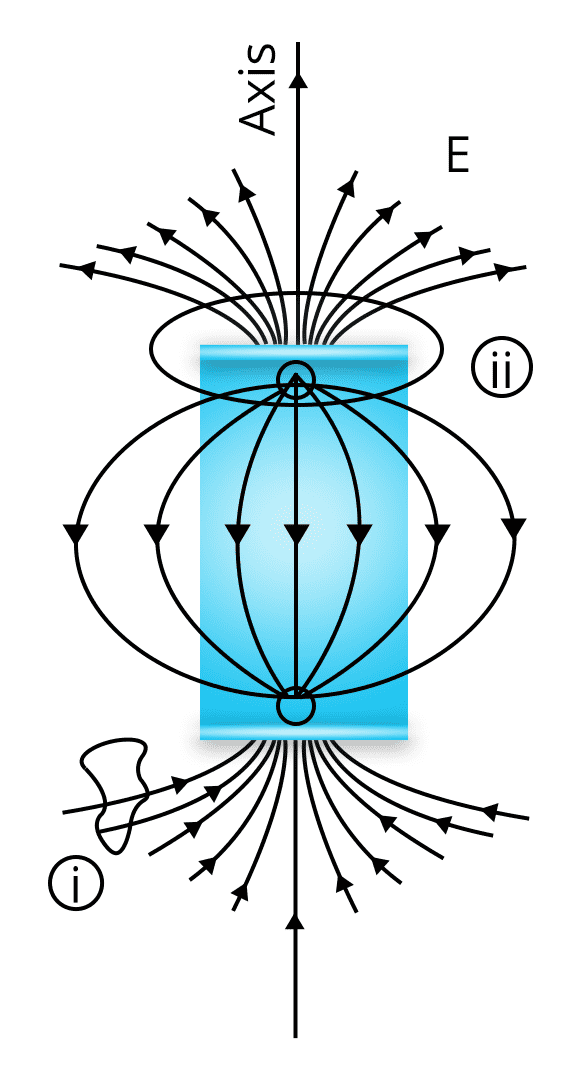4. Ohm’s law Ohm’s law is a basic principle used in study of eleectrodynamics or current electricity.The law gives a relationship between the voltage, current and resistance offered.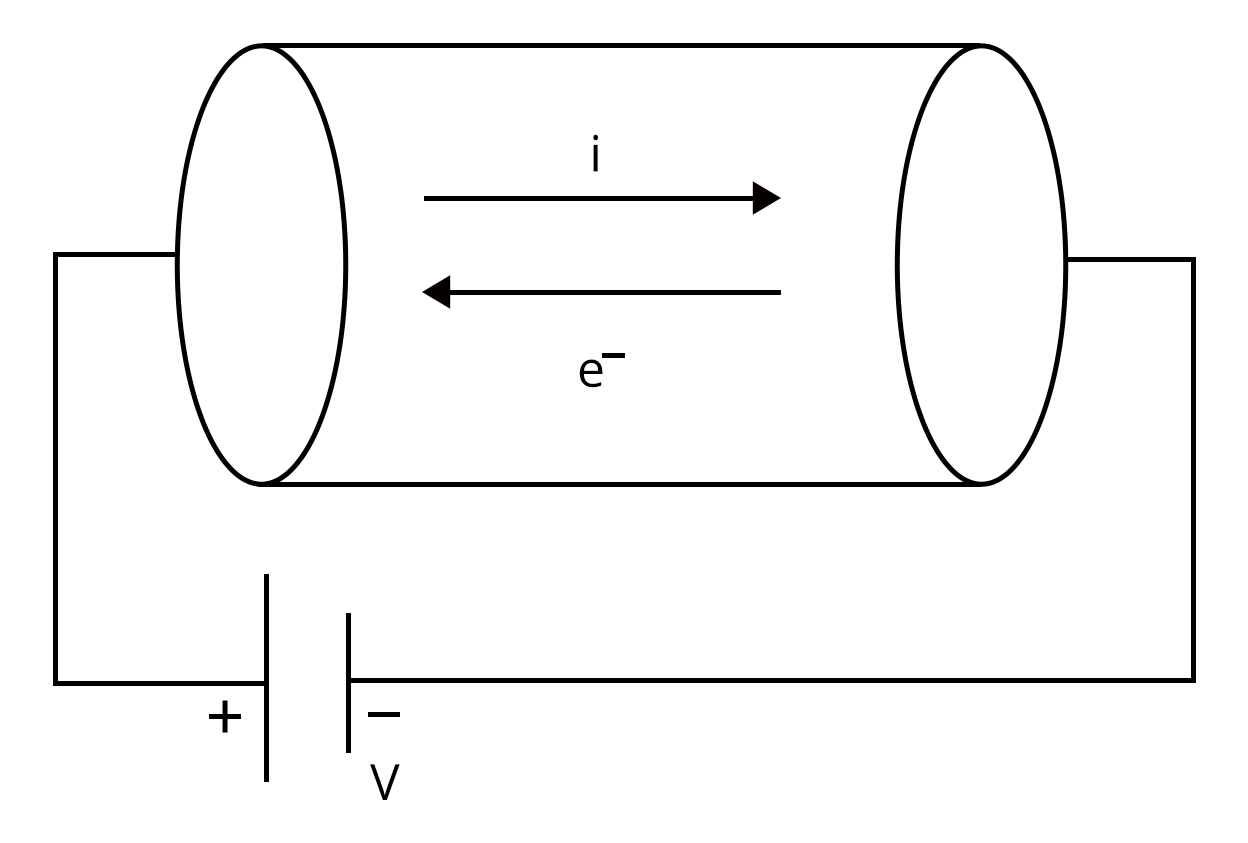Mathematically stated as:$V \propto I$ i.e., The voltage applied in the circuit will be directly proportional to the current flowing in the circuit. 5. Kirchhoff's Current Law and Voltage Law Current law: The total current entering the node must be equal to the total current leaving the node.I.e., Iin=IoutVoltage law: In any closed circuit, the total voltage will be equal to the sum of the voltage drop within the circuit.I.e., V=V1+V2+... 6. Fleming’s Right Rule If we arrange our thumb, forefinger and middle finger of the right-hand perpendicular to each other, then The thumb points towards the direction of the motion of conductor relative to the magnetic field.The middle finger points towards the direction of the induced current.The forefinger points towards the direction of the magnetic field.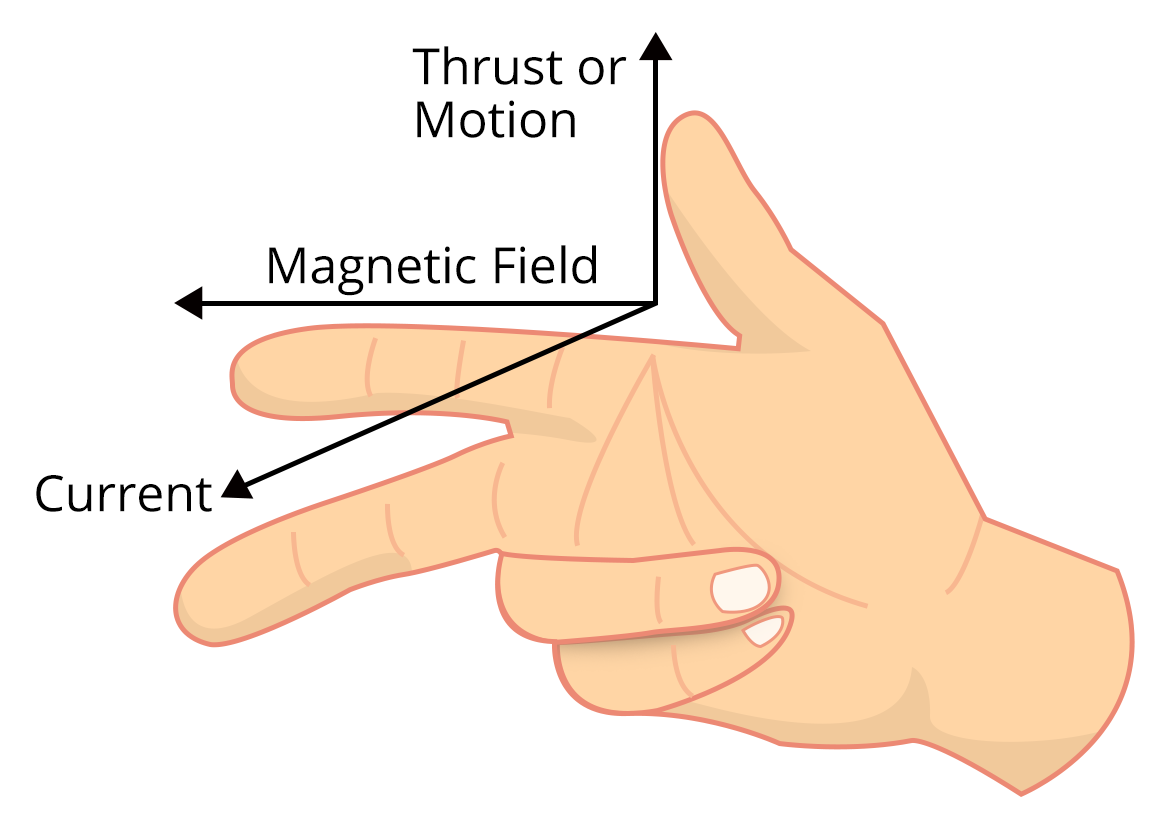7. Fleming’s Left Rule If we arrange our thumb, forefinger and middle finger of the left-hand perpendicular to each other, then The thumb points towards the direction of the force experienced by the conductor.the forefinger points towards the direction of the magnetic field. The middle finger points towards the direction of the electric current.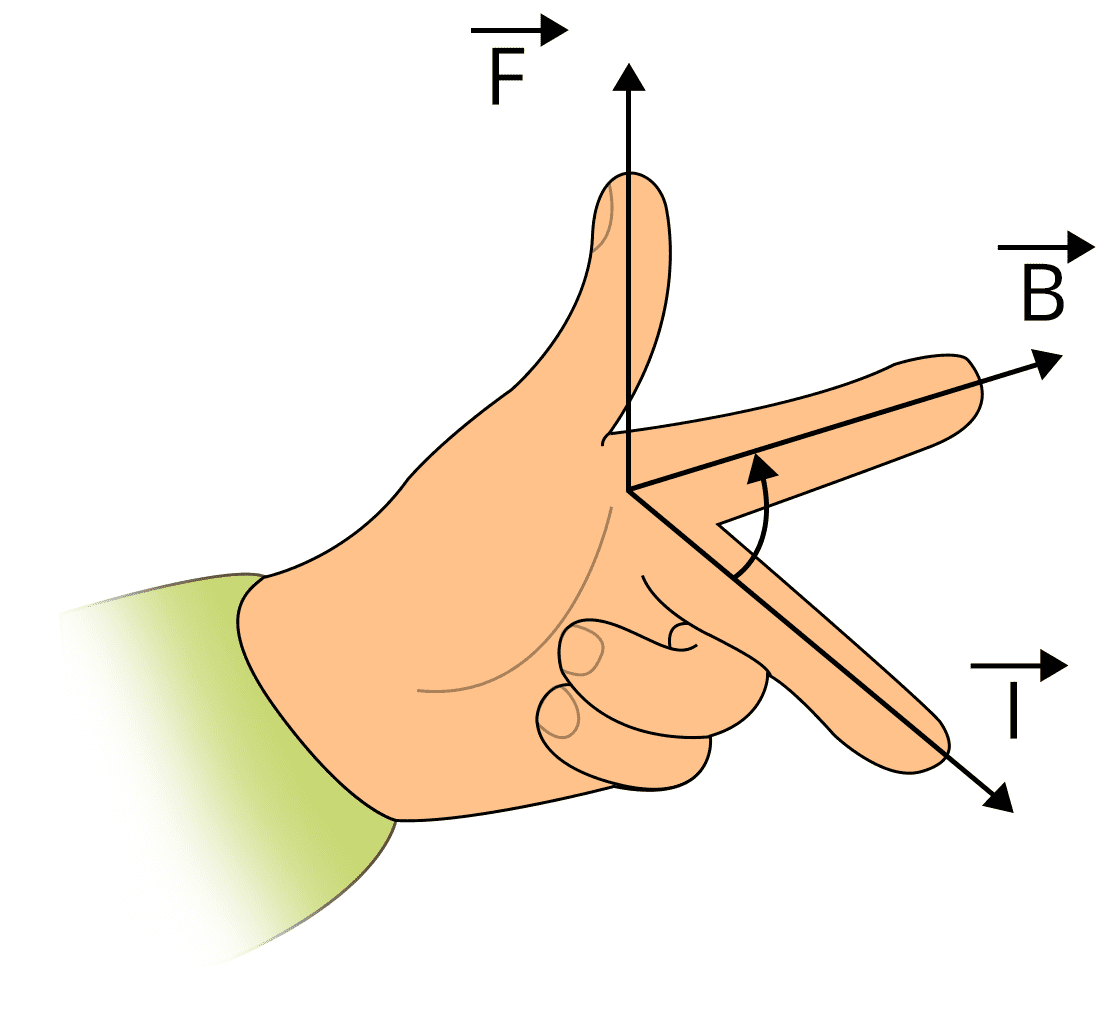8. Biot Savart’s Law It states that: “the magnetic field due to a tiny current element at any point is proportional to the length of the current element, the current, the sine of the angle between the current direction and the line joining the current element and the point, and inversely proportional to the square of the distance of that point. The direction of the magnetic field is in the direction of dl cross r.” 9. Ampere’s law Statement: “The magnetic field created by an electric current is proportional to the size of that electric current with a constant of proportionality equal to the permeability of free space.” 10. Magnetic force Magnetic force is given by $\vec F= q\left(\vec v\times \vec B\right)$ 11. Hysteresis Loop If we take a ferromagnetic material in completely demagnetized state and make it to undergo through a cycle of magnetization in which H is increased from zero to a maximum value Hmax, then decreases to zero, then reversed and again taken to –Hmax, and finally brought back to zero.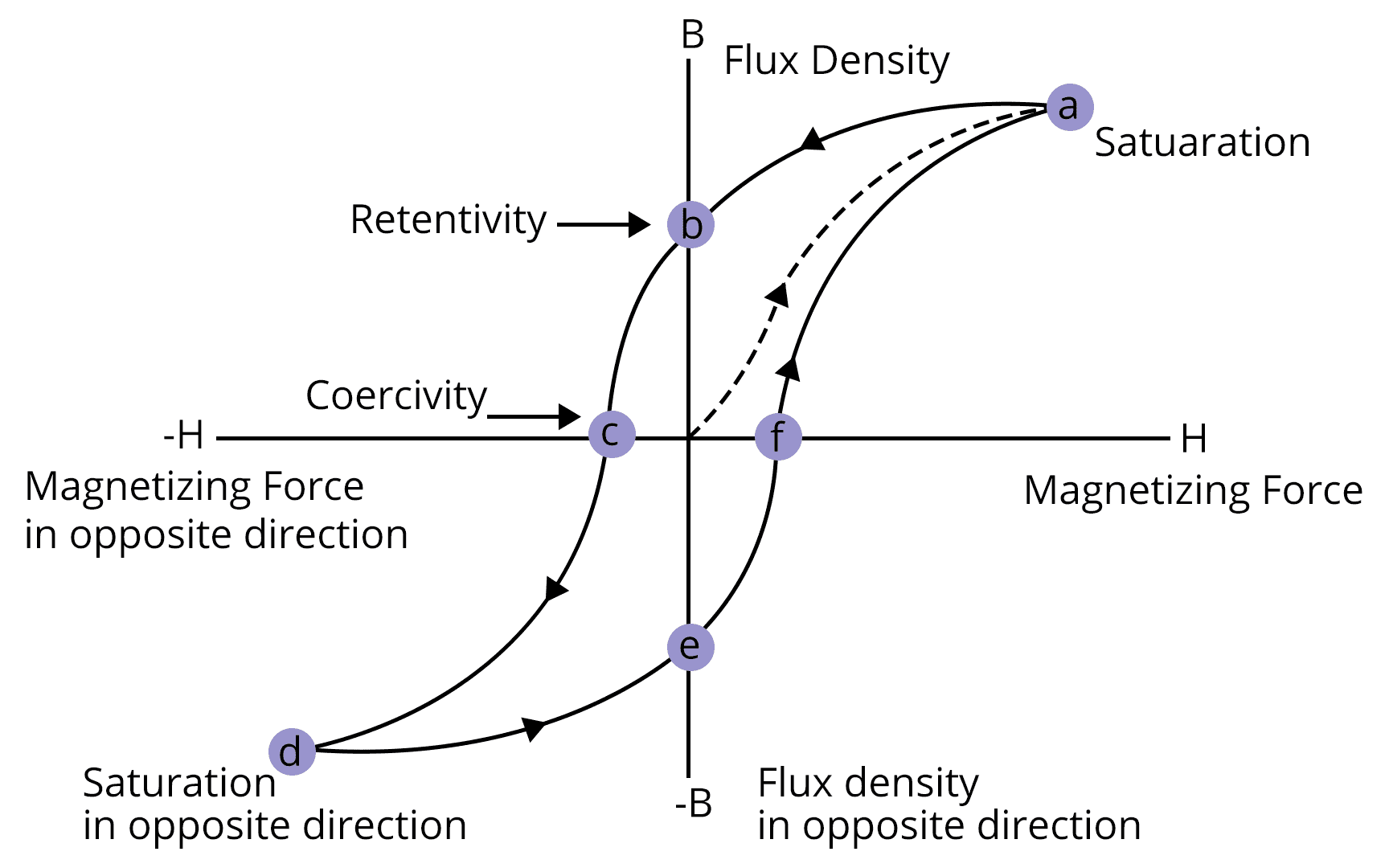12. Magnetic substances Pramagnetic: The substances which when placed in a magnetic field acquires a feeble magnetization in the same sense as the applied fieldDiamagnetic: The magnetic substances which can be easily magnetised are known as diamagnetic substances. 13. Magnetic Induction Magnetic induction is the process by which an ordinary piece of iron briefly develops magnetic characteristics due to the presence of another magnet close by. 14. Electromagnetic waves Electric field of electro magnetic waves:E(x,t) = Emaxcos(kx - ωt + φ)Where,φ-The phase differenceMagnetic field of electro magnetic waves:B(x,t) = Bmaxcos(kx - ωt + φ)Where,φ-The phase difference

### List of Important Formulae of Electricity and Magnetism

 Sl. No. Name of Concept Formula 1. Electrostatic Force $\vec F=\dfrac {k {q_1}{q_2}}{r^2}$Where, r- The distance between two charges 2. Electric Field $\vec E=\dfrac {F}{q}$Where,F- The electrostatic force 3. Gauss law $\phi=\dfrac {q}{\epsilon_o}$ 4. Electric Potential $V=\dfrac {kq}{r}$Where, r- The distance between two charges 5. Ohm’s Law $V=IR$Where,R- The resistance of the resistors 6. Combination of Resistors Resistors in Series:${R_s}={R_1}+{R_2}+....$Resistors in Paralell:${R_p}=\dfrac {{R_1}{R_2}}{{R_1}+{R_2}}$ 7. Capacitors and Capacitance Capacitance of the capacitor:$C=\dfrac {q}{V}$Combination of capacitors:Series:${C_s}=\dfrac {{C_1}{C_2}}{{C_1}+{C_2}}$Parallel:${C_p}={C_1}+{C_2}+....$ 8. Electric field in parallel plate capacitor $E=\dfrac {\sigma}{\epsilon_o}=\dfrac {Q}{{\sigma_o}A}$ 9. Dielectric constant of a substance $k=\dfrac {C}{C_o}$ 10. Energy stored in capacitor $W=\dfrac {1}{2} QV$ 11. Biot savarts law $dB=\dfrac {\mu_o}{4\pi} \dfrac {I\times dl\times \sin \theta}{r^2}$ 12. Lorentz Force $F=q\left [\vec E+ \left (\vec v\times \vec B\right)\right ]$ 13. Amperes law $\int B.dl={\mu_o}I$ 14. Magnetic dipole $\vec {P_m}=I \vec A$ 15. Magnetic sucesptility $\chi =\dfrac {I}{H}$ 16. Tangent law $B=H \tan \theta$

### Solved Examples of Current Electricity and Magnetism

1. A particle of mass m and charge q moves with a constant velocity v along the positive x direction. It enters a region containing a uniform magnetic field B directed along the negative z direction, extending from x = 3 to x = 5. The minimum value of v required so that the particle can just enter the region.

Sol:

Given,

The mass of the particle=m

Charge of the particle=q

The particle is moving along positive x axis with constant velocity v directed along the negative z axis. Now, we are asked to determine the minimum value of v, for entering the field B.

In this problem, the magnetic field B is perpendicular to the motion of particle the particle in the given region.

Therefore,

The magnetic force=The centripetal force

$qv B=\dfrac {m{v^2}}{r}$

$v=\dfrac {qBr}{m}$

Here, the region is extended from x=3 to x=5, thus the value of r will be:

r=5-3=2

Thus, we get:

$v=\dfrac {2qB}{m}$

Hence, the minimum value of v, for entering the field B is $\dfrac {2qB}{m}$.

Key Point: In this problem we can note one important formula i.e., the minimum value of v when B is perpendicular to the motion of the particle will be $\dfrac {\left({x_2}-{x_3}\right)qB}{m}$.

2. A small circular loop of conducting wire has radius a and carries current I. It is placed in a uniform magnetic field B perpendicular to its plane such that when rotated slightly about its diameter and released, it starts performing simple harmonic motion of time period T. If the mass of the loop is m then :

1. $T=\sqrt {\dfrac {2m}{IB}}$

2. $T=\sqrt {\dfrac {\pi m}{IB}}$

3. $T=\sqrt {\dfrac {\pi m}{2IB}}$

4. $T=\sqrt {\dfrac {2\pi m}{IB}}$

Sol:

Given that, we have a loop of mass m and radius a, carrying a current I. The current loop is placed in field perpendicular to the magnetic field B.

Now, let us assume that the loop is slightly rotated by an angle $\theta$, then the torque acting on the loop will be:

$\tau = - MB \sin \theta$

$I\alpha=-MB \theta$ (Because, the angle $\theta$ is very small, thus $\sin \theta=\theta$)

$\dfrac {mr^2}{2}\alpha=- \left(\pi {r^2}I\right)B \theta$

$\alpha=\dfrac {2IB\pi}{m}\theta$.....(1)

We know that,

$\alpha = \omega^2 \theta$....(2)

From (1) and (2), we get:

$\omega^2 =\dfrac {2\pi IB}{m}$

$\omega = \sqrt{\dfrac {2\pi IB}{m}}$

Therefore, time period T of rotation will be:

$T=\dfrac {2\pi}{\omega}=\dfrac {2\pi}{\dfrac {2\pi IB}{m}}$

$T=\sqrt {\dfrac {2\pi m}{IB}}$

Therefore, option D is the right answer.

Key Point: To solve the questions like this we need to learn a few standard values of moment of inertia, to save our calculation time.

### Previous Year Questions of Electricity and Magnetism

1. A coil having N turns is wound tightly in the form of a spiral with inner and outer radii 'a' and 'b' respectively. Find the magnetic field at centre, when a current I passes through coil: (JEE 2021)

1. $\dfrac {{\mu_o}IN}{2\left(b-a\right)} \log_e \left(\dfrac {b}{a}\right)$

2. $\dfrac {{\mu_o}I}{8}\dfrac {a+b}{a-b}$

3. $\dfrac {{\mu_o}I}{4\left(a-b\right)} \left[\dfrac {1}{a}-\dfrac {1}{b}\right]$

4. $\dfrac {{\mu_o}I}{8}\dfrac {a-b}{a+b}$

Sol:

Let us consider a small element dr at a distance r. Then the number of turns in the coil will be:

$dN=\dfrac {N}{b-a} dr$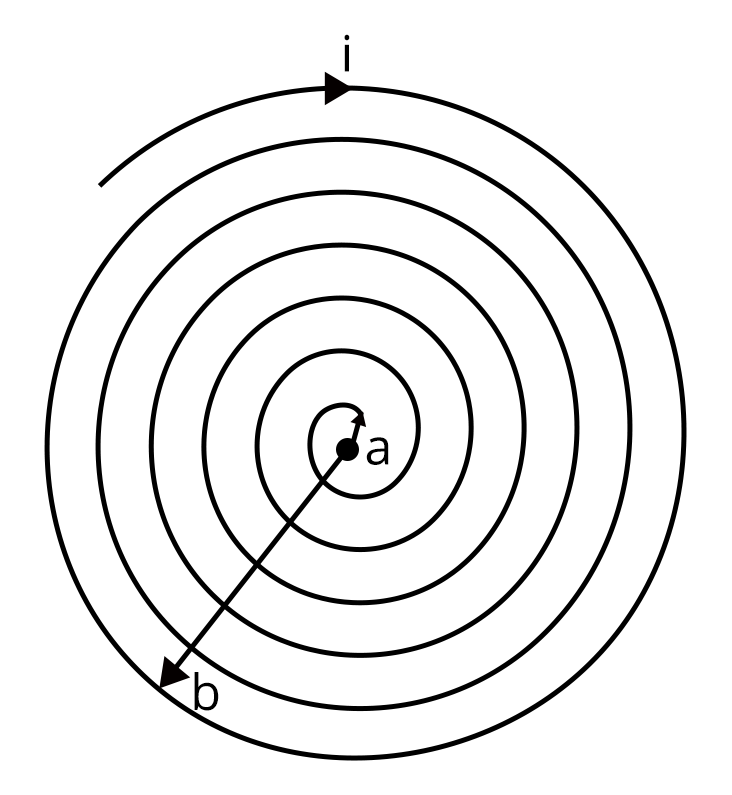Then the magnetic field due to the element dr at the center of the coil will be:

$dB=\dfrac {{\mu_o}I}{2r} dN$

$dB=\dfrac {{\mu_o}I N}{2 r\left (b-a)\right)}$

$\int_{r=a}^{r=b} dB=\int_{r=a}^{r=b}\dfrac {{\mu_o}I N}{2 r\left (b-a)\right)}$

$B=\dfrac {{\mu_o}I N}{2 \left (b-a)\right)} \log_e \dfrac {b}{a}$

Therefore, option A is the right answer.

Trick: The problem can be easily solved by looking at the the integration part, among all the option, only option A was having logarithmic value.

2. Following plots show magnetization (M) vs magnetizing field (H) and magnetic susceptibility () vs temperature (T) graph : (JEE 2021)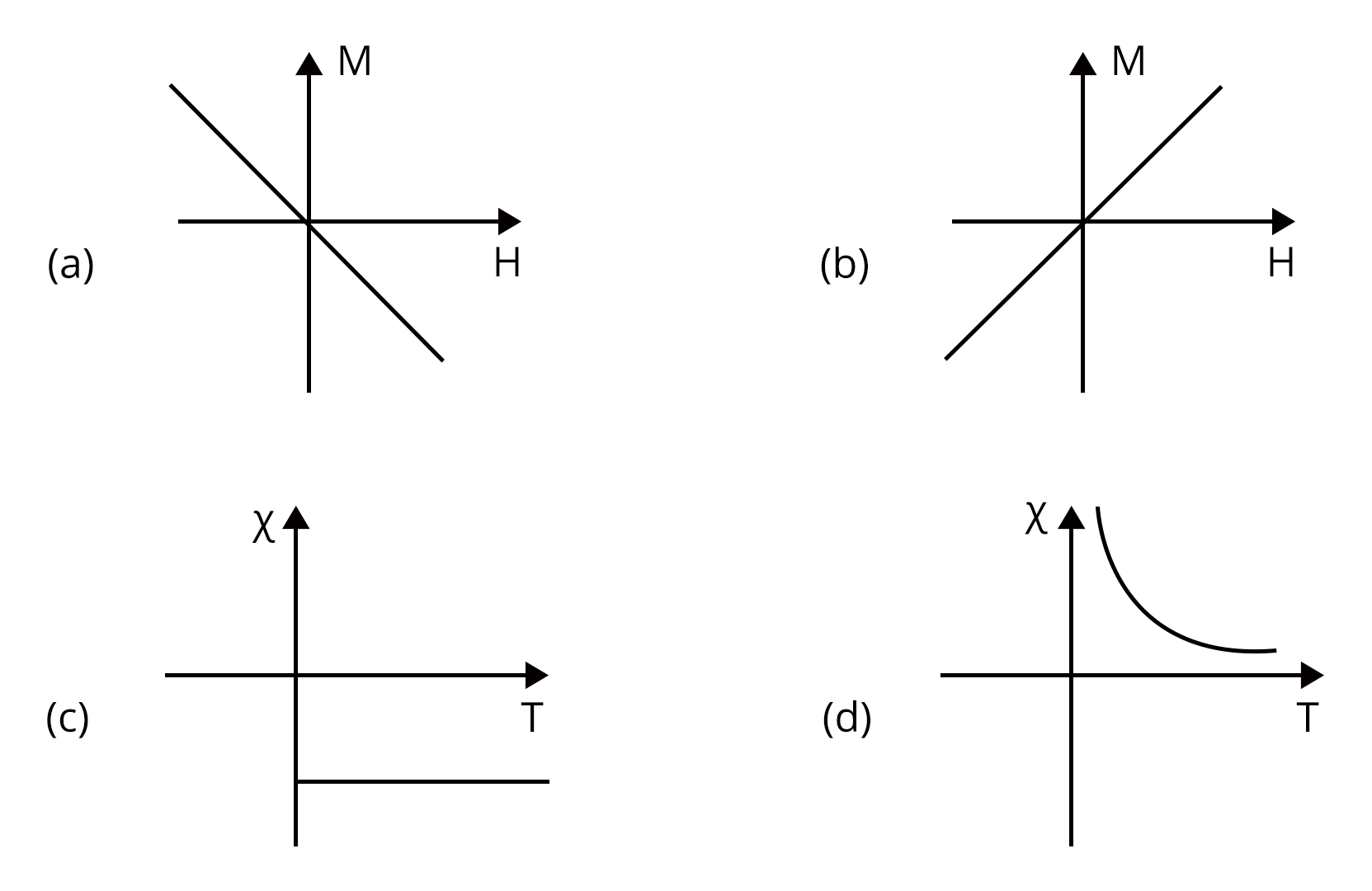1. (a), (c)

2. (a), (d)

3. (b), (c)

4. (b), (d)

Which of the following combination will be represented by a diamagnetic material?

Sol:

We know that susceptibility of diamagnetic materials will be negative and independent of temperature. From above graphs, graph (a) and graph (c) represents the diamagnetic material.

Therefore, option a is the right answer.

Trick: From graph eliminate the graphs that are having temperature dependency and porosity susceptibility.

### Practice Questions

1. Electric field of plane electromagnetic wave propagating through a non-magnetic medium is given by E = 20cos(2  1010 t-200x) V/m. The dielectric constant of the medium is equal to : (Take $\mu_r$ = 1) (Ans: 9)

2. A current of 1.5 A is flowing through a triangle, of side 9 cm each. The magnetic field at the centroid of the triangle is (Assume that the current is flowing in the clockwise direction.) (Ans: $3\times {10^{-5}}$ T, inside the plane of triangle)

### Conclusion

In this article we had an overview of the unit electricity and magnetism which is having many subtopics. In the article we discussed a few important concepts of electricity and magnetism in exam point of view. We have also discussed about the list of important formulae along with solved examples, previous year question, etc…

See MoreView all JEE Advanced Exam Dates
JEE Advanced 2023 exam date have been announced by the NTA. JEE Advanced 2023 will now be conducted on 4-June-2023, and the exam registration closes on 7-May-2023. You can check the complete schedule on our site. Furthermore, you can check JEE Advanced 2023 dates for application, admit card, exam, answer key, result, counselling, etc along with other relevant information.
See More
View all JEE Advanced Exam DatesApplication Form
Eligibility Criteria
Reservation Policy
IIT Guwahati has announced the JEE Advanced 2023 application form release date on the official website https://jeeadv.ac.in/. JEE Advanced 2023 Application Form is available on the official website for online registration. Besides JEE Advanced 2023 application form release date, learn about the application process, steps to fill the form, how to submit, exam date sheet etc online. Check our website for more details.View JEE Advanced Syllabus in Detail
It is crucial for the the engineering aspirants to know and download the JEE Advanced 2023 syllabus PDF for Maths, Physics and Chemistry. Check JEE Advanced 2023 syllabus here along with the best books and strategies to prepare for the entrance exam. Download the JEE Advanced 2023 syllabus consolidated as per the latest NTA guidelines from Vedantu for free.
See More
View JEE Advanced Syllabus in Detail## JEE Advanced 2023 Study Material

View all study material for JEE Advanced
JEE Advanced 2023 Study Materials: Strengthen your fundamentals with exhaustive JEE Advanced Study Materials. It covers the entire JEE Advanced syllabus, DPP, PYP with ample objective and subjective solved problems. Free download of JEE Advanced study material for Physics, Chemistry and Maths are available on our website so that students can gear up their preparation for JEE Advanced exam 2023 with Vedantu right on time.
See More
All
Chemistry
Maths
Physics
See Allsee all
See MoreView all JEE Advanced Important Books
In order to prepare for JEE Advanced 2023, candidates should know the list of important books i.e. RD Sharma Solutions, NCERT Solutions, HC Verma books and RS Aggarwal Solutions. They will find the high quality readymade solutions of these books on Vedantu. These books will help them in order to prepare well for the JEE Advanced 2023 exam so that they can grab the top rank in the all India entrance exam.
See More
Maths
NCERT Book for Class 12 Maths
Physics
NCERT Book for Class 12 Physics
Chemistry
NCERT Book for Class 12 Chemistry
Physics
H. C. Verma Solutions
Maths
R. D. Sharma Solutions
Maths
R.S. Aggarwal Solutions
See AllView all mock tests
See MoreIIT Bombay is responsible for the release of the JEE Advanced 2023 cut off score. The qualifying percentile score might remain the same for different categories. According to the latest trends, the expected cut off mark for JEE Advanced 2023 is 50% for general category candidates, 45% for physically challenged candidates, and 40% for candidates from reserved categories. For the general category, JEE Advanced qualifying marks for 2021 ranged from 17.50%, while for OBC/SC/ST categories, they ranged from 15.75% for OBC, 8.75% for SC and 8.75% for ST category.
See MoreSee More
Rank List
Counselling
Cutoff
JEE Advanced 2022 State Wise Rank List - The respective state authorities started releasing the JEE Advanced 2022 state merit lists for admission to Engineering course. Check JEE Advanced 2022 state-wise rank list for the year 2022 here.View all JEE Advanced 2023 Top Colleges
Want to know which Engineering colleges in India accept the JEE Advanced 2023 scores for admission to Engineering? Find the list of Engineering colleges accepting JEE Advanced scores in India, compiled by Vedantu. There are 1622 Colleges that are accepting JEE Advanced. Also find more details on Fees, Ranking, Admission, and Placement.
See More## FAQs on JEE Important Chapter - Electricity and Magnetism

FAQ

1. Is electricity and magnetism important for JEE?

In terms of complexity and weightage, 'Electricity and Magnetism' is one of the most important topics in the JEE syllabus. You can be confident of a high overall score if you have a firm grasp on this subject.

2. What is the weightage of electricity and magnetism in JEE Mains?

Due to its vast syllabus electricity and magnetism bagged high weightage in exam. Nearly 5-6 questions have been asked repeatedly which carry about 15-20 marks.

3. Is electricity and magnetism chapters tough?

No, both the chapters are interrelated to each other. Hence if we prepare thoroughly for electricity part, we can easily understand magnetism concepts. So these are not tough chapters.

4. Who unified electricity and magnetism?

Unification of electricity and magnetism credit goes to physicist James Clerk Maxwell.29 May 20231 days remaining04 Jun 20237 days remainingJEE (Advanced) 2023 Examination Paper 1
04 Jun 20237 days remainingJEE (Advanced) 2023 Examination Paper 2
04 Jun 20237 days remainingOnline display of provisional answer keys
11 Jun 202314 days remainingArchitecture Aptitude Test (AAT) 2023
21 Jun 202324 days remainingDeclaration of results of AAT 2023
24 Jun 202327 days remaining## Notice board

JEE News
JEE BlogsTrending pages